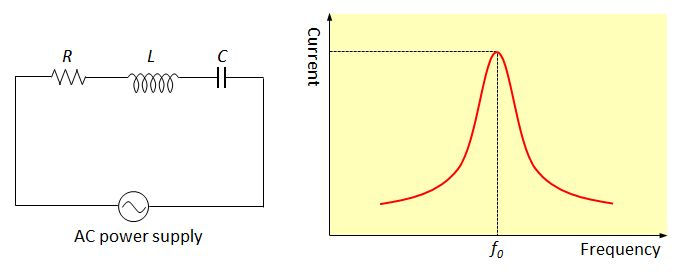# Resonance frequencyThe above left is an RLC series circuit, where the resistance of the resistor, the self-inductance of the inductor and the capacitance of the capacitor are $R$, $L$ and $C$, respectively. The above right shows the current intensity as a function of the frequency of the AC power supply, where $f_0$ is the resonance frequency of this circuit. Which of the following methods can make the resonance frequency greater than $f_0$?

a) Replacing the inductor with one that has an inductance greater than $L.$
b) Replacing the capacitor with one that has a conductance greater than $C.$
c) Connecting more capacitors with capacitance $C$ in series to the existing capacitor.

×